# Math Algebra Solver Calculator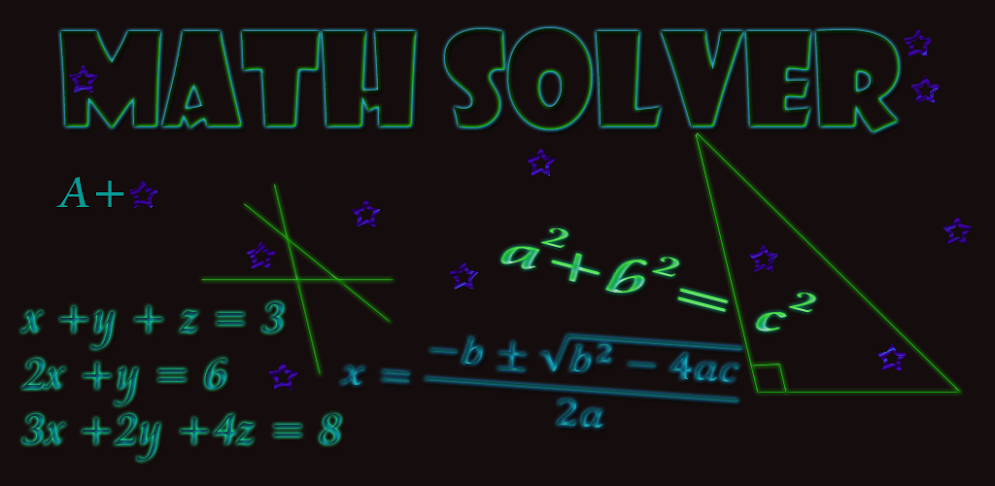## Description

This is a algebra calculator that solves algebra for you, and gives you answers teachers will accept! Unlike other similar apps this app is built directly for students. The answers that appear will be in the form your teacher will want. No more weird decimal answers!

Finally an app with answers teachers will accept! This does a variety of algebra calculations to help with your math class. Its like your own personal algebra tutor app. This is great for students in all algebra classes including algebra 2 it will act as a algebra 2 calculator and calculator for all algebra classes. It will act as a calculator to solve algebra problems like Factoring and complete the square.

Also Including quadratic equation solver, system of linear equations with two or three equations. pythagorean theorem, with two versions, one for simple problems and one for more advanced problems. It will also solve slope, y-intercept and give the equation in slope intercept form.

Simplifying square roots, and is a calculator that calculates square roots, cubed roots and any other root. We have added the foil method and exponents to the list of problems that can be solved. Reduce, add, subtract, divide and mixed number fractions. Finding LCM and GCD (least common multiple and greatest common divider).

There are many more solvers included in this app, as well as a list of formulas! This is great for kids and adults its, it can be used to help with math workout and math games. Free updates are constantly being released to improve the app based on your responses! Download now for this special price!

## Screenshots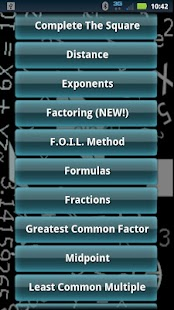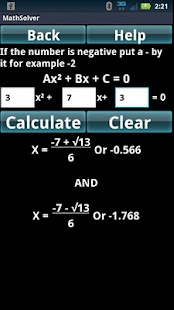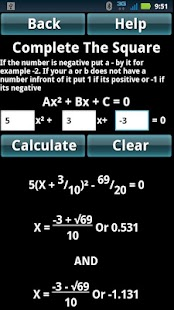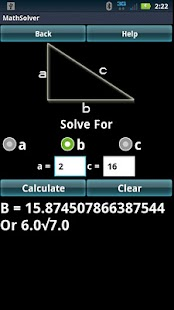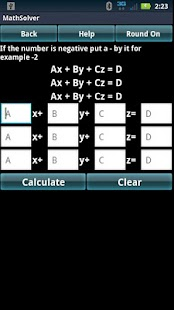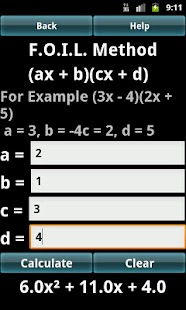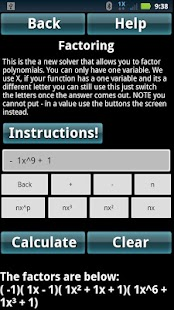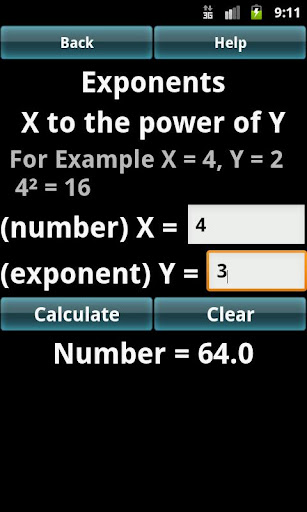Version 2.3 Uploader Hoster headhuntaz mediafire.comDownload nexus mediafire.comDownload